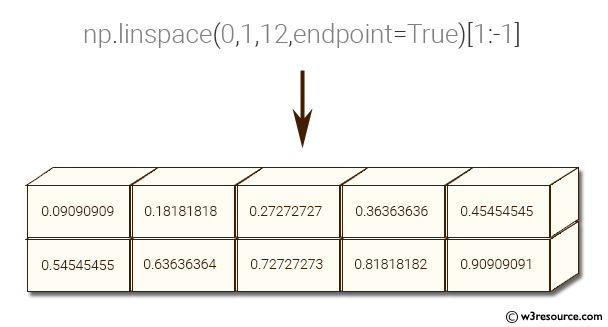﻿ NumPy: Create a vector of size 10 with values ranging from 0 to 1, both excluded - w3resource# NumPy: Create a vector of size 10 with values ranging from 0 to 1, both excluded

## NumPy: Array Object Exercise-66 with Solution

Write a NumPy program to create a vector of size 10 with values ranging from 0 to 1, both excluded.

Pictorial Presentation:Sample Solution:-

Python Code:

``````import numpy as np
x = np.linspace(0,1,12,endpoint=True)[1:-1]
print(x)
```
```

Sample Output:

```[ 0.09090909  0.18181818  0.27272727  0.36363636  0.45454545  0.5454545
5
0.63636364  0.72727273  0.81818182  0.90909091]
```

Python Code Editor:

Have another way to solve this solution? Contribute your code (and comments) through Disqus.

What is the difficulty level of this exercise?

Test your Python skills with w3resource's quiz

﻿

## Python: Tips of the Day

Set comprehension:

```>>> m = {x ** 2 for x in range(5)}
>>> m
{0, 1, 4, 9, 16}
```